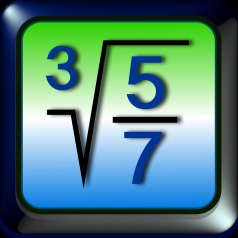iMathics

# Buttons

Reset Button

With the reset button one returns to the basic frame. The zoom scale is set to 1:1. The input mathematical expression is displayed at the left of the display screen.

Cursor shift buttons

The cursor can be moved with this two buttons to the right or left.

Shift Button

(only on the iPhone or iPod touch)

With the shift button one can switch between the standard keyboard and the scientific keyboard.

Delete Button

Pressing this button the item on the left of the cursor is deleted.

Clear Button

Pressing this button the whole content of the display screen is deleted.

Memory Clear Button

Pressing this button the content of the Memory is deleted.

Memory Recall Button

Pressing this button the content of the Memory is inserted in the current expression.

Pressing this button the value of the current expression is evaluated and added to the memory.

Fraction Button

A new fraction is inserted. For a mixed fraction, first type in the whole part, then press the fraction button for inserting the fraction part.

Slash Button

A fraction bar is inserted. The number immediatly before the slash will be the numerator, the number after the slash is the denominator of the newly created fraction.

Root Button

With the root button one can input a square root, cubic root or generally a root with an arbitrary exponent. To type in the exponent, place the cursor in the upper left corner of the root sign.

Degree Button

Pressing the degree button, an angle is measured in degrees, minutes and seconds instead of radians. The degree button is important by trigonometric functions. By the functions sin, cos and tan it indicates that the argument is measured in degrees; by the functions arcsin, arccos and arctan it indicates that the result is calculated in degrees.

Equal Button

If you press the equal button the input expression is evaluated. The result is displayed as a decimal number.

Equal Mixed Fraction Button

If you press this button the input expression is evaluated. The result is displayed as a mixed fraction. If the result is not a rational number, it is displayed as a decimal number.

Equal Fraction Button

If you press this button the input expression is evaluated. The result is displayed as a simple fraction. If the result is not a rational number, it is displayed as a decimal number.

Equal Degree Button

If you press this button the input expression is evaluated. The result is displayed in degrees/minutes/seconds